Courses

# Percentage And Its Applications Quant Notes | EduRev

## Quantitative Techniques for CLAT

Created by: Gyanm Institute

## Quant : Percentage And Its Applications Quant Notes | EduRev

The document Percentage And Its Applications Quant Notes | EduRev is a part of the Quant Course Quantitative Techniques for CLAT.
All you need of Quant at this link: Quant

Chapter - 11

PERCENTAGE AND ITS APPLICATIONS

‘Per cent’ means ‘out of one hundred’. Symbol % is used to denote per cent

Some examples to convert a per cent into a fraction, ratio or decimal and vice versa.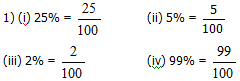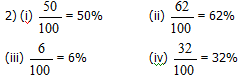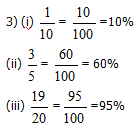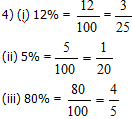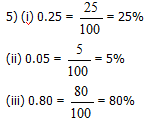6) (i) 50% = 0.50

(ii) 7% = 0.07

(iii) 90% =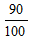= 0.90

(iv) 1% = 0.01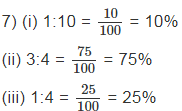8) (i) 10% of 100 = 10

(ii) 20% of 500 = 100

(iii) 30% of 1000 = 300

9) (i) 5 out of 25 = 20%

(ii) Re 1 out of Rs 25 = 4%

(iii) 10 out of 50 = 20%

Solved examples:

(i) If 25% of X= 30 find X

Sol. 25% of X is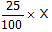and it is equal to 30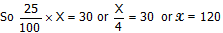(ii) If 4.6% of X is 23 find X

Sol. 4.6% of X means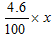and it is given to be equal to 23

So= 23

or X =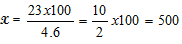(iii) If 24% of X is 48, then find x

Sol. 24% of X is 48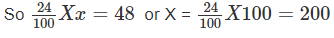Example 2: Vijay deposits Rs 45 per month in the bank. If this amount is 15% of his monthly income, find his monthly income.

Solution: Let his monthly income be Rs X

As per the given statement 15% of X is 45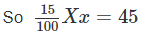or x =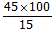= 300 RS

Example 3: Gunpowder contains 75% nitre and 10% sulphur. The rest of the material is charcoal. Find the amount of each of the contents in 9 kg of gunpowder.

Solution: Weight of gunpowder = 9 kg

Contents are – nitre = 75%, Sulphur = 10% charcoal = 100-75-10 = 15%

Nitre in 9 kg =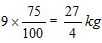= 6.75 kg.

Sulphur in 9 kg =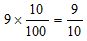= 0.90 kg.

Charcoal in 9 kg =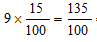= 1.35 kg.

Example 4: Priya got 87.5% marks in the annual examination. If she got 700 marks, find the total number of marks in the examination.

Solution: Let total marks be X

A per the statement 87.5% of X = 700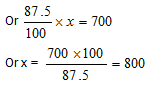So total marks = 800

Example 5: The value of a machine depreciates by 10% every year. If its present value is Rs 38700, what was its value one year ago.

Solution: Let the value last year be x

If it is decreased by 10% the present value will be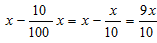and as per the given statement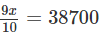or x =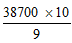= 4300 X 10 = Rs. 43000.

Example 6: The salary of a person has been increased by 25%. By what per cent should the salary be reduced so that he gets the original salary.

Solution: Let original salary be 100

Salary after an increase of 25% = 100+25=125

To restore the salary back to 100 it should be decreased by Rs 25

The reduction on 125 = Rs 25

The reduction on 100 =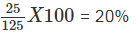Example 7: Ankits salary is 25% more than that of Anil. What percent is Anil’s salary less than Ankits?

Solution: Let Anil’s salary be Rs 100

Then Ankit gets 25% more than Anil = 125

Now      If Ankit’s salary is Rs 125 Anil’s = 100.

If Ankit’s salary is Rs 100 then Anil’s =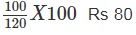So Anils salary is Rs 80 when Ankit’s is Rs 100

So Anil salary is 20% less than Ankit

Example 8: Raman loses 20% of his money. After spending 25% of the remaining, he has Rs 480. How much did he originally had?

Solution: Suppose Raman had Rs 100 in the beginning. He lost 20% i.e. Rs. 20

Remainder = Rs 100 – Rs 20 = Rs 80

Out of Rs 80 he spent 25%, which is equal to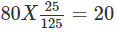Remainder = Rs 80 – Rs 20 = Rs 60

If remainder is Rs 60, he originally had = Rs 100

If remainder is Rs1, he originally had =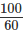If remainder is Rs 480 he originally had =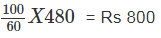Example 9: The price of wheat increases by 10%. By what per cent should a consumer reduce his consumption so that his expenditure of wheat remains the same.

Solution: Let the original consumption of wheat is 100 kg and the price is Rs 100 per kg.

Expenditure originally = Rs 100 x 100

Expenditure now if consumption is X kg @ Rs 110 per kg = x X 110 Rs

But the expenditure remains to be the same

So x X 110 = 100 X 100

Or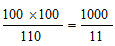So reduction in consumption =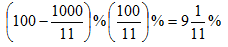Example 10: The price of a shirt in Dec 1997 was Rs 250. In Jan. 1998 it was increased by 10% and in Oct 1998 it was reduced by 10%. What in the new price of the shirt.

Solution: Price in Dec 1997 = Rs 250

Price in Jan 1998 =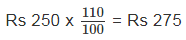Price in Oct 1998 = 275 x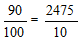= Rs 247.50

Example 11: In an examination a candidate must secure 40% marks to pass. A candidate got 220 marks and failed by 20 marks. What are the maximum marks for the examination.

Solution: Let the maximum marks be x

Marks required to pass =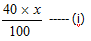As per the statement the marks required to pass = 220 +20 = 240 ------- (ii)

So from I and II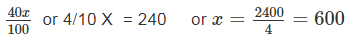Example 12: When 75% of a number is added to 75, the result is the same number. Find the number.

Solution: Let the number be x

As per the given statement.

75% of X + 75 = x

Or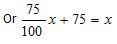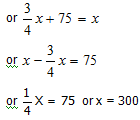## Quantitative Techniques for CLAT

63 videos|22 docs|93 tests

,

,

,

,

,

,

,

,

,

,

,

,

,

,

,

,

,

,

,

,

,

;